Select Page

Share

The SUBTOTAL function is designed for columns of data, or vertical ranges. It is not designed for rows of data, or horizontal ranges. According to Microsoft, it is generally easier to create a list with subtotals by using the Subtotal command in the Outline group on the Data tab in the Excel desktop application. Once the subtotal list is created, you can modify it by editing the SUBTOTAL function.

An example of this usage is in the following simple illustration.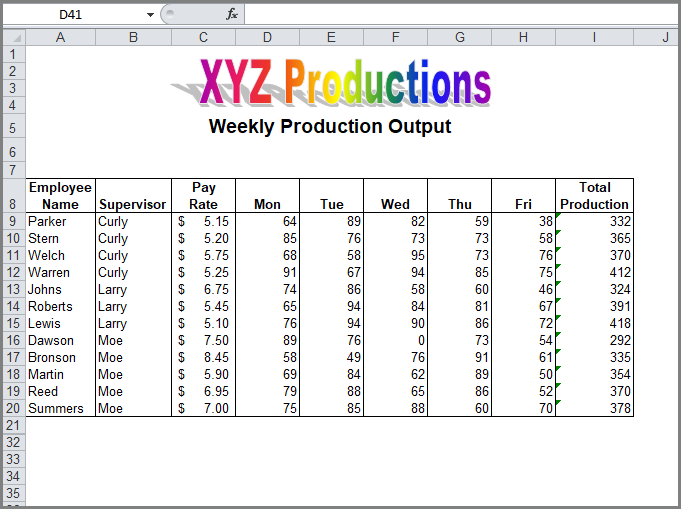In the Supervisor column (Column B) there are three separate values: Curly, Larry, and Moe. If you wanted Excel to calculate summaries at every change in Supervisor, you could apply the Subtotal feature. Place your cursor in any cell within the table and on the Data tab, click on Subtotal in the Outline group.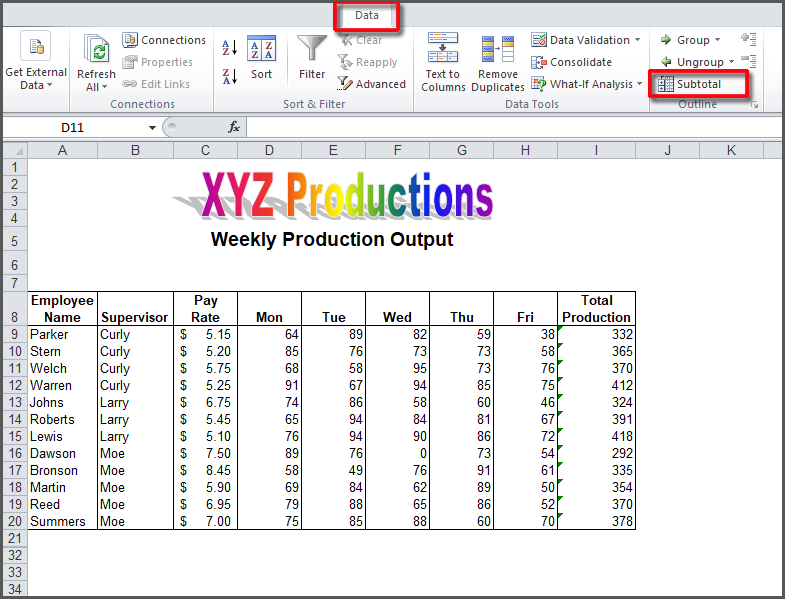Excel will use the column headings of your data and you will choose one of them (like Supervisor in this example) to define where the breaks will be. You will be asked to define what statistic you wish to use to summarize the data (Sum, Count, Average, etc.) and which columns you want subtotals for.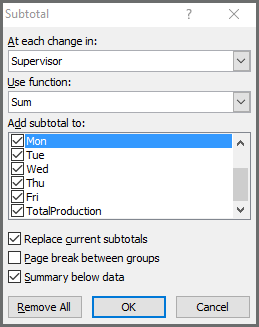With the settings defined here, this is the result. Notice that Excel calculated totals at each change in Supervisor. Notice that to use this feature, the table must be sorted correctly.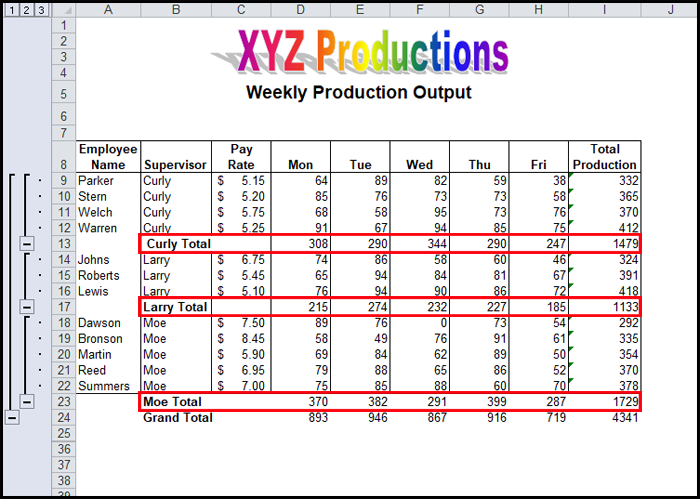While the subtotal feature is active, Excel displays a pane to the left that shows three Outline viewing levels:

1 displays the grand total row

2 displays the subtotal rows

3 displays everything

You can click on level 2 to hide the detail rows and display only the subtotals.Click on 3 to display all the rows again.

If you look at any of the subtotals, you will see that Excel inserted the SUBTOTAL function.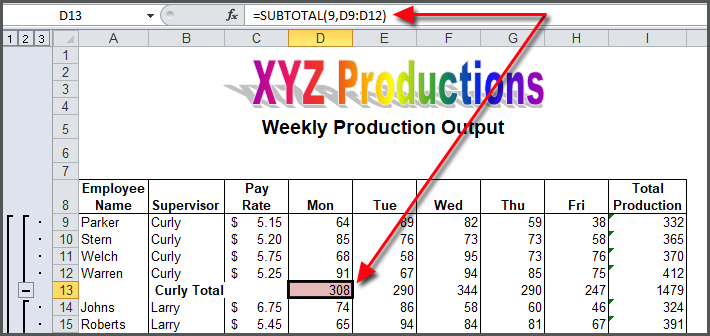The syntax is as follows:

SUBTOTAL(function_num,ref1,[ref2],…)

The following table defines the function_num and references.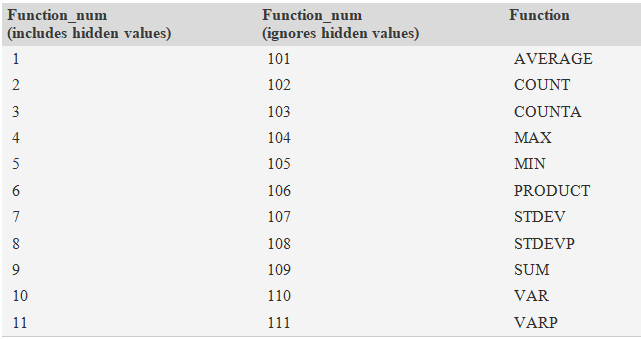You can see that it’s easier to use the Subtotal feature from the Data tab and have Excel insert the functions for you.

Share